Question

# A baseball of mass 0.15 kg is thrown at a batter at a speed of 42...

A baseball of mass 0.15 kg is thrown at a batter at a speed of 42 m/s, and, after being hit, travels in the opposite direction.

If the final speed of the baseball is 35 m/s and the baseball and the bat are in contact for 2 ms, what is the average force the bat applies to the baseball?

What percentage of the original kinetic energy is lost?

for the average force

average force on the ball = change in momentum/time taken

average force on the ball = m * (v - u)/time

average force on the ball = 0.15 * (35 - (-42))/0.002

average force on the ball = 5775 N

the average force on the ball is 5775 N

----

percentage of original kinetic energy lost = (-0.50 *m * v^2 + 0.50 *m * u^2)/(0.50 * m * u^2)

percentage of original kinetic energy lost = (42^2 - 35^2)/42^2

percentage of original kinetic energy lost = 0.306 = 30.6 %

the percentage of original kinetic energy lost is 30.6 %

#### Earn Coins

Coins can be redeemed for fabulous gifts.

Similar Homework Help Questions
• ### A bsebal approaching a batter with a speed of 31 m/s is hit and leaves the...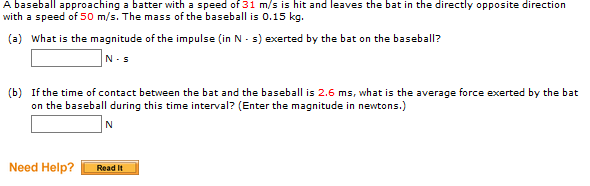A bsebal approaching a batter with a speed of 31 m/s is hit and leaves the bat in the directly opposite direction with a speed of 50 m/s. The mass of the baseball is 0.15 kg. What is the ma gnitude of the impulse (in N s) exerted by the bat onthebaseball? N-S (b) If the time of contact between the bat and the baseball is 2.6 ms, what is the average force exerted by the bat on the baseball...

• ### A batter swings at a baseball (mass 0.145 kg) that is moving horizontally toward him at...

A batter swings at a baseball (mass 0.145 kg) that is moving horizontally toward him at a speed of 40.0 m/s. He hits a line drive with the ball moving away from him horizontally at 50.0 m/s just after it leaves the bat. Part A If the bat and ball are in contact for 8.00 ms, what is the average force that the bat applies to the ball? Express your answer with the appropriate units.

• ### A pitcher throws a 0.143-kg baseball toward the batter so that it crosses home plate horizontally...

A pitcher throws a 0.143-kg baseball toward the batter so that it crosses home plate horizontally and has a speed of 42 m/s just before it makes contact with the bat. The batter then hits the ball straight back at the pitcher with a speed of 51 m/s. Assume the ball travels along the same line leaving the bat as it followed before contacting the bat. (a) What is the magnitude of the impulse delivered by the bat to the...

• ### A pitcher throws a 0.138-kg baseball toward the batter so that it crosses home plate horizontally...

A pitcher throws a 0.138-kg baseball toward the batter so that it crosses home plate horizontally and has a speed of 42 m/s just before it makes contact with the bat. The batter then hits the ball straight back at the pitcher with a speed of 46 m/s. Assume the ball travels along the same line leaving the bat as it followed before contacting the bat. (a) What is the magnitude of the impulse delivered by the bat to the...

• ### What is the final velocity of the baseball?

A 0.145 kg baseball is thrown at a speed of 35 m/s. The batter strikes the ball with a force of 25,000N. The bat and ball are in contact for 0.500 ms.Please be clear with the steps.

• ### A 0.15 kg baseball travelling horizontally is hit by a baseball bat. The velocity of the...

A 0.15 kg baseball travelling horizontally is hit by a baseball bat. The velocity of the baseball changes from +2-m/s to -20m/s as a result of the contact. a) what is the magnitude of the impulse delivered to the ball? b) If the ball is in contact with the bat for 1.20msec, what is the average force delivered by the bat? c)If the instantaneous force delivered by the bat increases linearly for 0.65 ms and then decreases linearly for 0.65ms,...

• ### A baseball with a mass of 137 g is thrown horizontally with a speed of 40.4...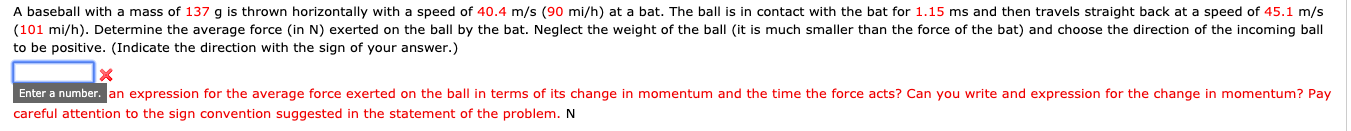A baseball with a mass of 137 g is thrown horizontally with a speed of 40.4 m/s (90 mi/h) at a bat. The ball is in contact with the bat for 1.15 ms and then travels straight back at a speed of 45.1 m/s (101 mi/h). Determine the average force in N) exerted on the ball by the bat. Neglect the weight of the ball (it is much smaller than the force of the bat) and choose the direction of...

• ### A baseball with a mass of 141 g is thrown horizontally with a speed of 40.1...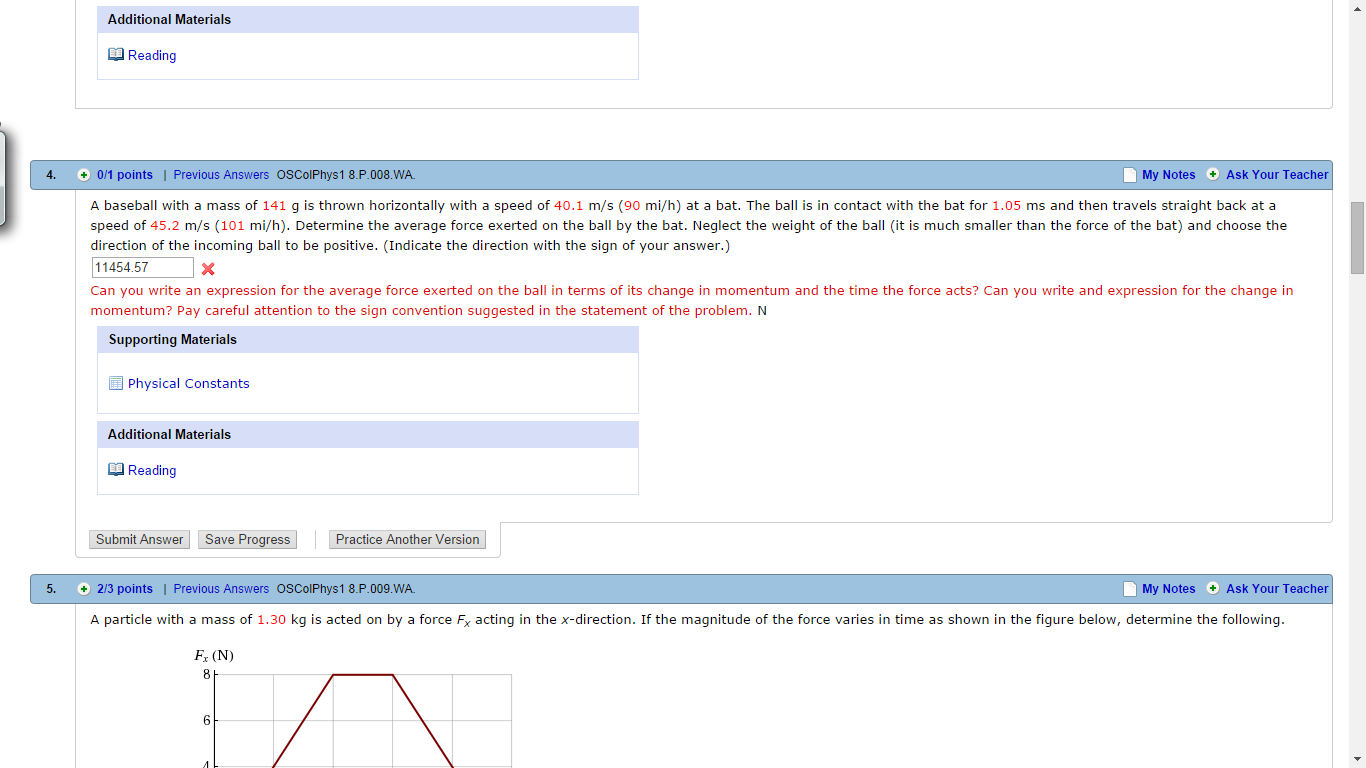A baseball with a mass of 141 g is thrown horizontally with a speed of 40.1 m/s (90 mi/h) at a bat. The ball is in contact with the bat for 1.05 ms and then travels straight back at a speed of 45.2 m/s (101 mi/h). Determine the average force exerted on the ball by the bat. Neglect the weight of the ball (it is much smaller than the force of the bat) and choose the direction of the incoming...

• ### A baseball with a mass of 152 g is thrown horizontally with a speed of 40.7...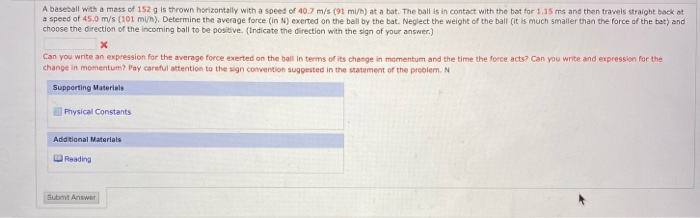A baseball with a mass of 152 g is thrown horizontally with a speed of 40.7 m/s (91 mi/h) at a bat. The ball is in contact with the bat for 1.15 ms and then travels straight back at a speed of 45.0 m/s (101 mi/h). Determine the average force (in N) exerted on the ball by the bat. Neglect the weight of the ball (it is much smaller than the force of the bat) and choose the direction of...

• ### A baseball approaches home plate at a speed of 40.0 m/s, moving horizontally just before being...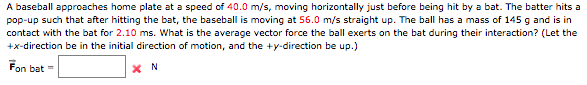A baseball approaches home plate at a speed of 40.0 m/s, moving horizontally just before being hit by a bat. The batter hits a pop-up such that after hitting the bat, the baseball is moving at 56.0 m/s straight up. The ball has a mass of 145 g and is in contact with the bat for 2.10 ms. What is the average vector force the ball exerts on the bat during their interaction? (Let the +x-direction be in the initial...